# 1 & 2 persons - Page

Viewing mode
25 results
•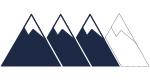• Slopes access :
• 50 -300m to the slopes
• Rooms :
• Studio
• Studio + cabin(s)
• Total surface (sq.m) :
• 20 sq.m
•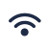•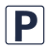•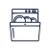•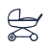•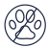•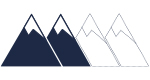• Slopes access :
• ski-in (-50 m)
• Rooms :
• 2 rooms
• Total surface (sq.m) :
• 21 sq.m
••••• Slopes access :
• 50 -300m to the slopes
• Rooms :
• Studio
• Total surface (sq.m) :
• 16 sq.m
••••••• Slopes access :
• 50 -300m to the slopes
• Rooms :
• Studio
• Total surface (sq.m) :
• 17 sq.m
••••• Slopes access :
• 50 -300m to the slopes
• Rooms :
• 2 rooms
• Total surface (sq.m) :
• 27 sq.m
••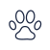•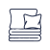••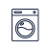•• Slopes access :
• 50 -300m to the slopes
• Rooms :
• Studio + cabin(s)
• Total surface (sq.m) :
• 20 sq.m
•••••• Slopes access :
• 50 -300m to the slopes
• Rooms :
• Studio
• Total surface (sq.m) :
• 19 sq.m
•••• Slopes access :
• 50 -300m to the slopes
• Rooms :
• Studio
• Studio + cabin(s)
• Total surface (sq.m) :
• 20 sq.m
•••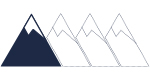• Slopes access :
• ski-in (-50 m)
• Rooms :
• Studio
• Studio + cabin(s)
• Total surface (sq.m) :
• 20 sq.m
•••• Slopes access :
• 50 -300m to the slopes
• Rooms :
• Studio
• Studio + cabin(s)
• Total surface (sq.m) :
• 20 sq.m
••••• Slopes access :
• 50 -300m to the slopes
• Rooms :
• Studio
• Total surface (sq.m) :
• 18.91 sq.m
•••••• Slopes access :
• 50 -300m to the slopes
• Rooms :
• Studio
• Total surface (sq.m) :
• 19 sq.m
•••• Slopes access :
• 50 -300m to the slopes
• Rooms :
• Studio
• Total surface (sq.m) :
• 19 sq.m
•••••• Slopes access :
• 50 -300m to the slopes
• Rooms :
• Studio
• Total surface (sq.m) :
• 17.17 sq.m
••• Slopes access :
• 50 -300m to the slopes
• Rooms :
• Studio
• Total surface (sq.m) :
• 16 sq.m
•23m2, Living room with pull out sofa bed Equipped kitchenette (vitroceramic hob, microwave / grill, dishwasher, ...
• Slopes access :
• ski-in (-50 m)
• Rooms :
• Studio
• Total surface (sq.m) :
• 23 sq.m
•••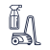•••• Slopes access :
• ski-in (-50 m)
• Rooms :
• Studio
• Total surface (sq.m) :
• 13 sq.m
••• Slopes access :
• 50 -300m to the slopes
• Rooms :
• Studio + cabin(s)
• Total surface (sq.m) :
• 17 sq.m
•••20m2, Living room with pull out bed for 2 people Equipped kitchenette (electric hob with 2 burners, fridge, microwave /...
• Slopes access :
• 50 -300m to the slopes
• Rooms :
• Studio
• Total surface (sq.m) :
• 20 sq.m
•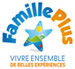•••••• Slopes access :
• ski-in (-50 m)
• Rooms :
• 2 rooms
• Total surface (sq.m) :
• 35 sq.m
•••